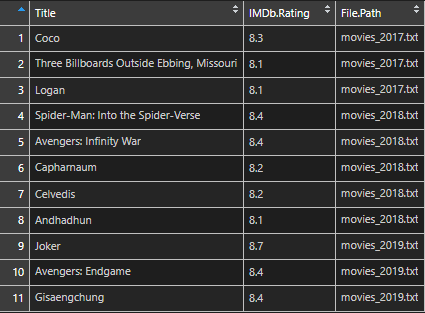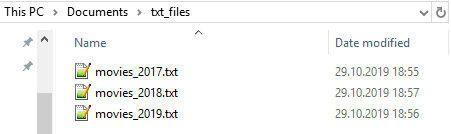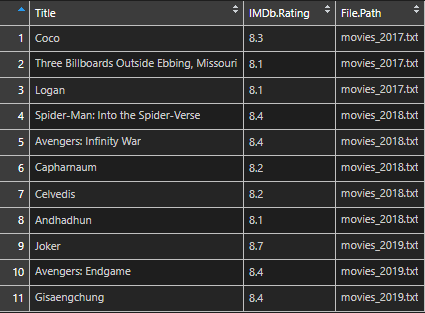# How to combine files with R and add filename columnHere is a simple way how to combine CSV or text files with R and, at the same time, add a column with filenames. Here is all the code with more detailed explanations below.

```require(dplyr)
require(data.table)

all_paths <-
list.files(path = "~/txt_files/",
pattern = "*.txt",
full.names = TRUE)

all_content <-
all_paths %>%
sep = "\t",
encoding = "UTF-8")

all_filenames <- all_paths %>%
basename() %>%
as.list()

# combine file content list and file name list
all_lists <- mapply(c, all_content, all_filenames, SIMPLIFY = FALSE)

# unlist all lists and change column name
all_result <- rbindlist(all_lists, fill = T)
# change column name
names(all_result) <- "File.Path"```

I have 3 txt files, and each of them contains Tab-delimited movie data from IMDB.## To combine files with R and add filename column, follow these steps.

```all_paths <-
list.files(path = "~/txt_files/",
pattern = "*.txt",
full.names = TRUE)```

```all_content <-
all_paths %>%
sep = "\t",
encoding = "UTF-8")``````all_filenames <- all_paths %>%
basename() %>%
as.list()```4. Combine file content list with filename list

`all_lists <- mapply(c, all_content, all_filenames, SIMPLIFY = FALSE)`5. Unlist result and do some finalization

```all_result <- rbindlist(all_lists, fill = T)
# change column name
names(all_result) <- "File.Path"```It is also possible to do that at once.

```# all process in one

all_txt <- rbindlist(mapply(
c,
(
list.files(
path = "~/txt_files/",
pattern = "*.txt",
full.names = TRUE
) %>%
lapply(
sep = "\t",
encoding = "UTF-8"
)
),
(
list.files(
path = "~/txt_files/",
pattern = "*.txt",
full.names = TRUE
) %>%
basename() %>%
as.list()
),
SIMPLIFY = FALSE
),
fill = T)```

Please, check other R related posts that might be interesting for you.

### 1 comment on “How to combine files with R and add filename column”

1.Sophie

Such a clear explanation! Thank you so much!